### Formula Constrained Optimization

 Formula Constrained Optimization is a specialized optimization option based on formula representation of a multilayer coating. Some formula parameters may be considered as unknown parameters, part of which are continuous variables changing within some limits, other parameters may be integer parameters having a predefined set of values. For example, a quarter-wave stack with n pairs of layers with another non-quarter wave stack with m pairs and QWOT thicknesses equal to a and b can be represented as (H L)^n (a H b L)^m We can consider some set of possible values for integers n and m, and for every possible combination of n and m OptiLayer will try to find optimal values of continuous parameters a and b, optimizing the design performance with respect to loaded targets. Another example may be a stack having period with varying optical thicknesses. Ultra-wide range high reflectors, chirp mirrors and other coatings can be designed in this way. Example. High reflector operating in the spectral range from 400 nm to 900 nm. Refractive indices of layer materials nH=2.35 and nL=1.45, glass substrate. Width of the first high reflection zone of a quarter wave mirror with the central wavelength $$\lambda_0$$ can be estimated with the help of a known formula: $$\Delta=\lambda_u-\lambda_l,\;\;\displaystyle\frac{\lambda_u}{\lambda_l}=\frac{\pi+\arccos(-\xi)}{\pi-\arccos(\xi)}$$ $$\displaystyle\xi=\frac{n_H^2+n_L^2-6 n_Hn_L}{(n_H+n_L)^2}$$ where $$\lambda_u$$ and $$\lambda_l$$ are upper and lower boundaries of the high reflection zone. If the central wavelength is 650 nm then the width of the high reflection zone of a quarter wave mirror is about 200 nm. In our design problem we need to cover a spectral range 400-900 nm, i.e. we need a high reflection zone of 500 nm. It means that we need to combine several quarter wave or near quarter wave stacks.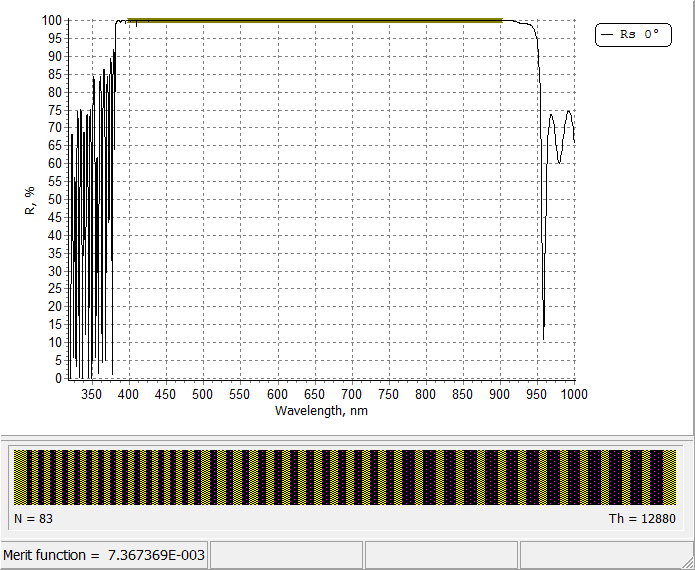Illustration of the design process of a wide band reflector. In order to observe the design process, put the mouse of the picture.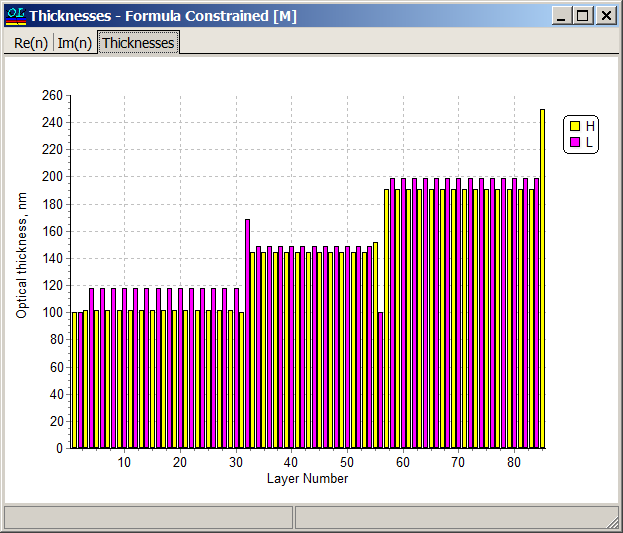Assuming that $$\lambda_l=400$$ nm and using formulas above, it is possible to estimate that three quarter wave stacks are required. The corresponding width of the high reflection zones are: $$\Delta_1=140 nm, \;\;\Delta_2=200 nm,\;\; \Delta_3=260 nm$$ Then we need three quarter wave or near quarter wave stacks and matching layers.  The design can be therefore represented by a formula: m1, m2, m3 are integer parameters indicating the number of pairs  a1, b1,... are continuous parameters expressing the fraction of quarter wave optical thickness. m1, m2, m3 can, for example, take values from 5 to 14. The parameters a1, b2,... can be varied in the segments [0.8;2] $$c_1 H \;d_1 L\; (a_1H \;b_1L)^{m_1} \;c_2H \;d_2L \;(a_2H\; b_2L)^{m_2}\; c_3H \;d_3L \;(a_3H\;b_3L)^{m_3} \;c_4H$$ In OptiLayer design formulas and their parameters can be specified in Synthesis --> Formula Constrained Optimization: OptiLayer will consider all possible combinations of specified integer parameters m1, m2, m3. Each of these parameters can take 10 values: 5, 6, ..., 14. For each m1, m2, m3 combination, OptiLayer optimizes the design with respect to continuous parameters a1, b1, c1,... All calculated design are stored in the "Design Collection Window". The designs are ranged with respect to the merit function values.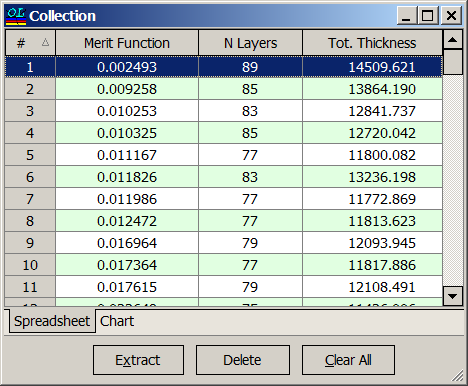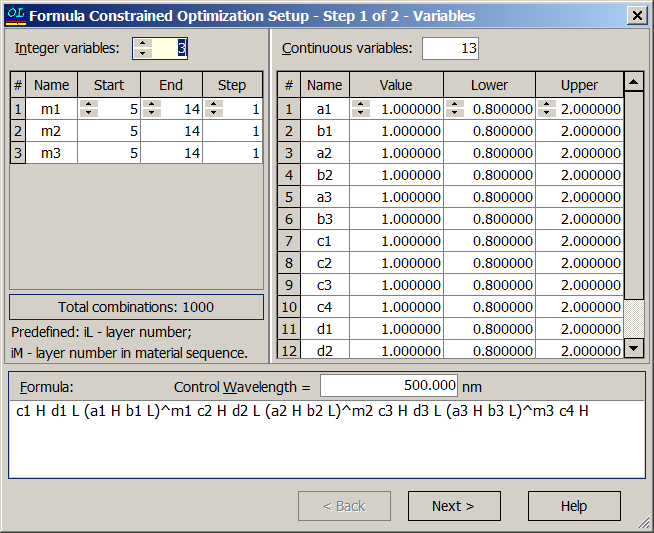This design example is illustrated in our video example "High Reflector Part II" on YouTube### Easy to startOptiLayer provides user-friendly interface and a variety of examples allowing even a beginner to effectively start to design and characterize optical coatings.        Read more...

### Docs / SupportComprehensive manual in PDF format and e-mail support help you at each step of your work with OptiLayer.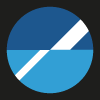If you are already an experienced user, OptiLayer gives your almost unlimited opportunities in solving all problems arising in design-production chain. Visit our publications page and challenge page.